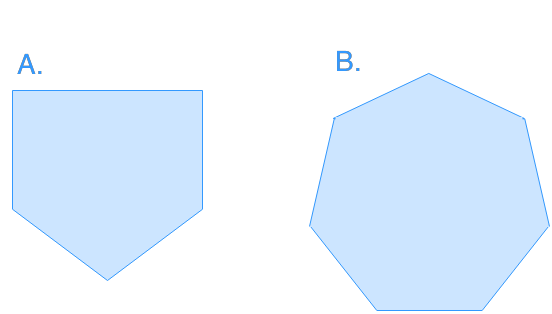Understanding tessellations

Understanding tessellations

The word tessellations may not be a familiar word to all of us, while tessellations are common in our everyday life. What exactly does it mean? Tessellations basically mean covering a surface without overlaps or gaps using a pattern of flat shapes. Using rectangular tiles to cover the kitchen floor is a good real life example of tessellations. We will learn more basic concepts of tessellations in this lesson.

Lessons

• Introduction
a)
How to find the sum of interior angles of a polygon?

b)
Tessellation
• What is tessellation?
• How to make a tessellation?

• 1.
Can you tessellate the plane with the following regular polygons? Explain.• 2.
Complete the table below.
 Polygon Interior Angle (°) Can it tessellate a plane? (Y/N) Equilateral Triangle Square Regular Pentagon Regular Hexagon Regular Heptagon